Brooks (Base) Square (BS) 101

~ The Architecture of Space-Time (TAOST)

&

The Conspicuous Absence of Primes (TCAOP) ~

I. TAOST / B. Geometrics - shapes

I. TAOST - the network

A. Geometrics – lines

B. Geometrics - shapes <---

C. Geometrics - relationships

1. Pythagorean

2. Parallelogram

3. Penta

II. TCAOP - everything minus the network

 TAOST: Rules 1-50 | Rules 51-80 | Rules 81-99 | Rules 100-107 | Rules 108-153 | TCAOP: Rule 154 | Rules 155-157 | Rules 158-159 | Rule 160 | Interconnectedness: Rules 161-175 | Appendix A: Rules 176-181 | Appendix B: Rules 182-200 |

I B. Geometrics - Shapes

There are two parts to this section on shapes. In the first part, we will be looking at shapes formed of diagonal lines within the grid. To illustrate these numerical relationships, we will be using the original Base Square (BS 1.00). The exact symmetry of the lower and upper triangles in the base version of the matrix grid show the patterns to the best advantage.

In the second part, individual geometric shapes will be the focus. For this part, we will revert back to the default Brooks Square (BS 1.01+) version.

Part 1- shaped by diagonals within the grid (BS 1.00).

s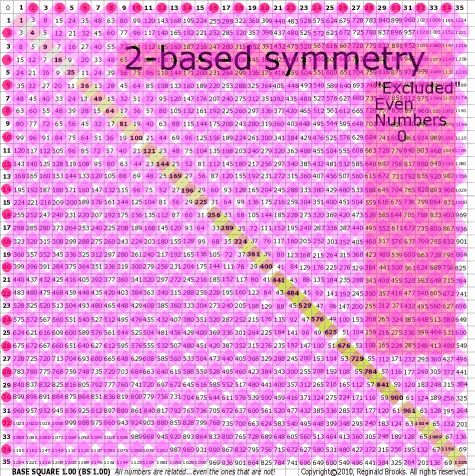~click to enlarge image 51 BS Rule 51: Linking all numbers whose value is a multiple of 2 generates a tight symmetrical gird of diamond-square shapes radiating outward from the Prime Diagonal (PD). Note: There are no pure link lines along the PD. Starting with 2, every other 2-based number is excluded from the Strict Inner Grid as: 2, 4, 6, 8, 10, 12, 14, 16, 18, 20, 22, 24, 26, 28, 30, 32, 34, 36, 38, 40, 42, 44, 46, 48, 50, 52, 54, 56, 58, 60, 62, 64, 66, 68, 70, 72, 74, 76, 78, 80, 82, 84, 86, 88, 90, 92, 94, 96, 98, 100, 102, 104, 106, 108, 110, 112, 114, 116, 118, 120, 122, 124, 126, 128, 130, 132, 134, 136, 138, 140, 142, 144, 146, 148, 150, ...and is the master exclusion list.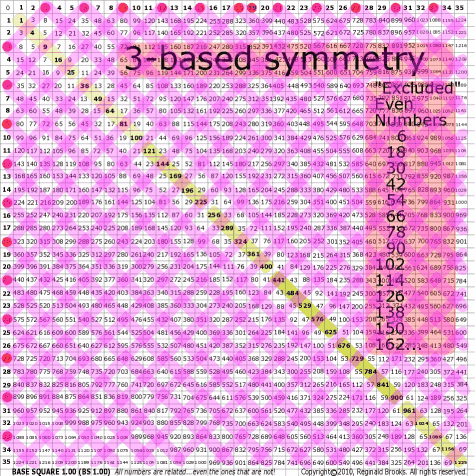~click to enlarge image 52 BS Rule 52: Linking all numbers whose value is a multiple of 3 generates a tight symmetrical gird of alternating hexagon-like shapes radiating outward from the Prime Diagonal (PD). Note: There are no pure link lines along the PD. Starting with 6, every fourth 3-based number is excluded from the Inner Grid as: 3, 6, 9, 12, 15, 18, 21, 24, 27, 30, 33, 36, 39, 42, ...

TOP ]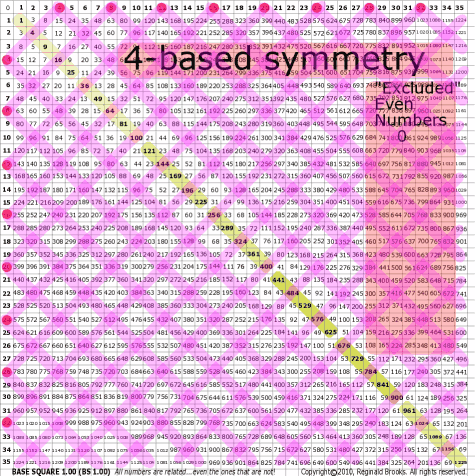~click to enlarge image 53 BS Rule 53: Linking all numbers whose value is a multiple of 4 generates a tight symmetrical gird of diamond-square shapes radiating outward from the Prime Diagonal (PD), overlapping the 2-based numbers, and revealing that all even numbers within the Inner Grid are divisible by 4. Note: There are no pure link lines along the PD. The same 2-based excluded numbers apply.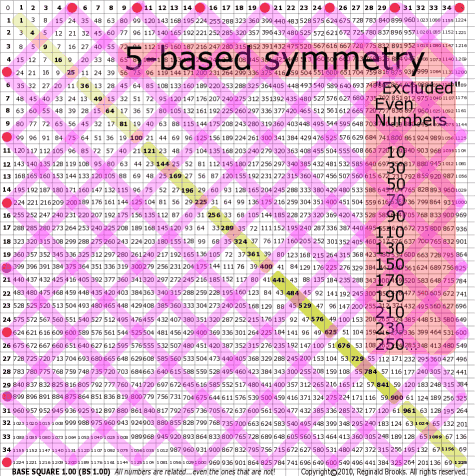~click to enlarge image 54 BS Rule 54: Linking all numbers whose value is a multiple of 5 generates a symmetrical grid of diamond-squares (3 numbers/side) radiating outward from the PD. Note: There are no pure link lines along the PD. Starting with 10, every fourth 5-based number is excluded from the Inner Grid: 5, 10, 15, 20, 25, 30, 35, 40, 45, 50, 55, 60,...

TOP ]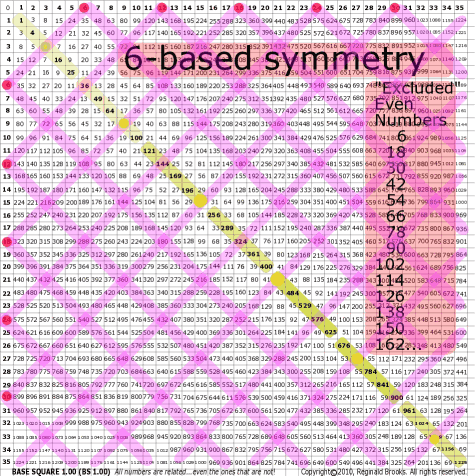~click to enlarge image 55 BS Rule 55: Linking all numbers whose value is a multiple of 6 generates a tight symmetrical gird of diamond-square shapes radiating outward from the Prime Diagonal (PD), overlapping numbers that are both 2- and 3-based. Note: Simply expands 3 x 2 = 6. There are no pure link lines along the PD. The same 2-based excluded numbers apply.

TOP ]

 TAOST: Rules 1-50 | Rules 51-80 | Rules 81-99 | Rules 100-107 | Rules 108-153 | TCAOP: Rule 154 | Rules 155-157 | Rules 158-159 | Rule 160 | Interconnectedness: Rules 161-175 | Appendix A: Rules 176-181 | Appendix B: Rules 182-200 |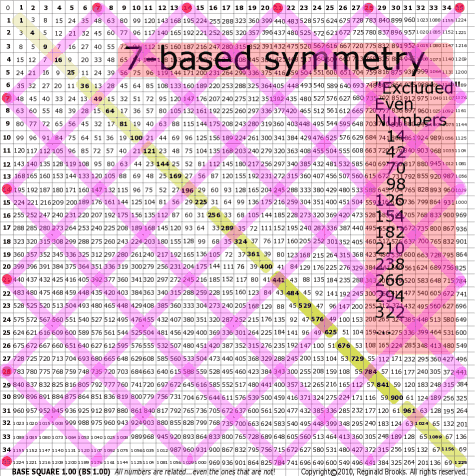~click to enlarge image 56 BS Rule 56: Linking all numbers whose value is a multiple of 7 generates a symmetrical gird of diamond-square shapes radiating outward from the Prime Diagonal (PD). Note: There are no pure link lines along the PD. Starting with 7, every fourth 7-based number is excluded from the Inner Grid as: 7, 14, 21, 28, 35, 42, 49, 56, 63, 70,...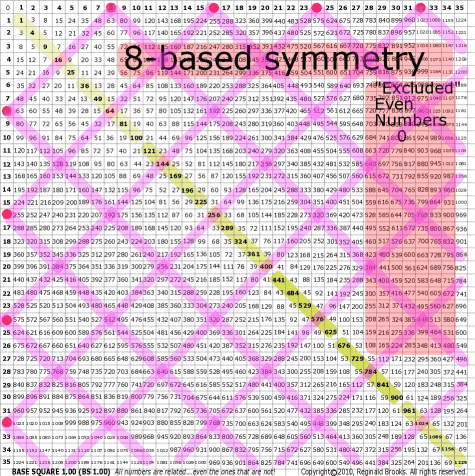~click to enlarge image 57 BS Rule 57:  Linking all numbers whose value is a multiple of 8 generates a symmetrical gird of diamond-square shapes radiating outward from the Prime Diagonal (PD) overlapping the 2-based numbers, and revealing that all even numbers within the Inner Grid are divisible by 4. Note: There are no pure link lines along the PD. The same 2-based excluded numbers apply.

TOP ]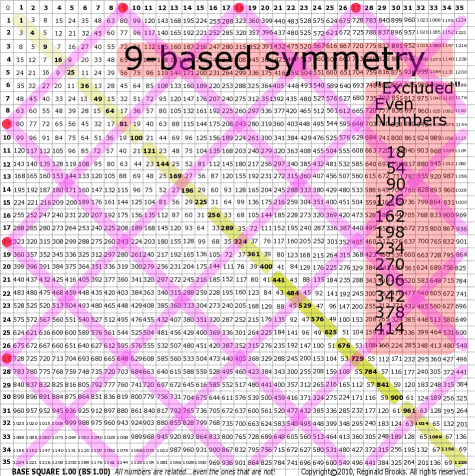~click to enlarge image 58 BS Rule 58: Linking all numbers whose value is a multiple of 9 generates a tight symmetrical gird of diamond-square shapes radiating outward from the Prime Diagonal (PD), overlapping numbers that are 3-based. Note: Simply expands 3 x 3 = 9. There are no pure link lines along the PD. The 9-based excluded Inner Grid numbers, a subset of the 2-based master exclusion list, are: 9, 18, 27, 36, 45, 54, 63, 72, 81, 90,....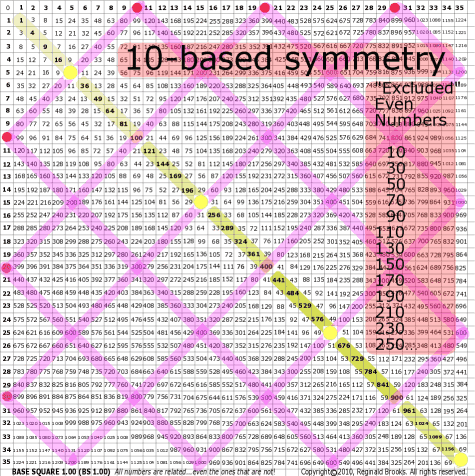~click to enlarge image 59 BS Rule 59: Linking all numbers whose value is a multiple of 10 generates a symmetrical grid of diamond-squares (3 numbers/side) radiating outward from the PD. Note: There are no pure link lines along the PD. Starting with 10, every other 10-based number is excluded from the Inner Grid: 10, 20, 30, 40, 50, 60,...

TOP ]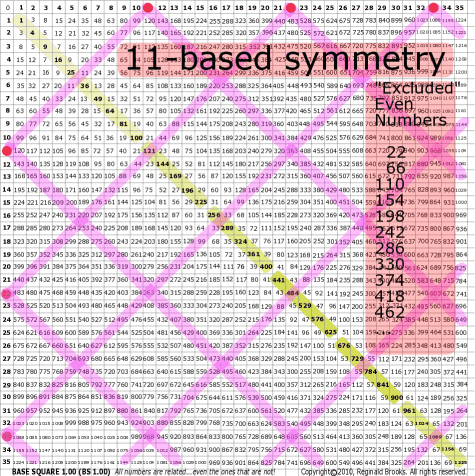~click to enlarge image 60 BS Rule 60:Linking all numbers whose value is a multiple of 11 generates a symmetrical gird of diamond-square shapes radiating outward from the Prime Diagonal (PD). And the so on for this series. Note: There are no pure link lines along the PD. Starting with 22, every fourth 11-based number is excluded from the Inner Grid as: 11, 22, 33, 44, 55, 66, 77, 88, 99, 110, 121, 132, 143, 154,...

TOP ]

 TAOST: Rules 1-50 | Rules 51-80 | Rules 81-99 | Rules 100-107 | Rules 108-153 | TCAOP: Rule 154 | Rules 155-157 | Rules 158-159 | Rule 160 | Interconnectedness: Rules 161-175 | Appendix A: Rules 176-181 | Appendix B: Rules 182-200 |

Part 2- individual shapes within the grid (BS 1.01+).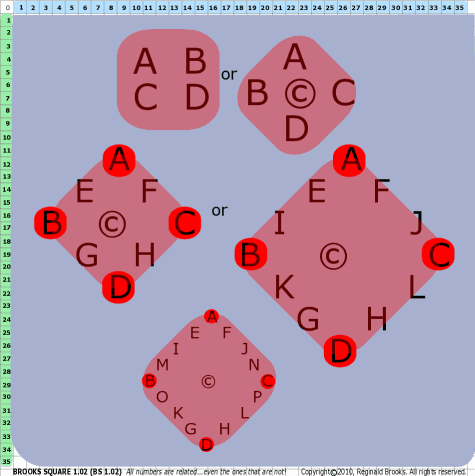Fig H. Standard geometric shapes ... like triangles, squares and rectangles (usually as diamond-shapes), pentagons, hexagons and octagons ... will have their constituent number values labeled in a somewhat symmetrical, book-reading format using “A”, “B”, “C”, “D”, ... as the perimeter and © as the center.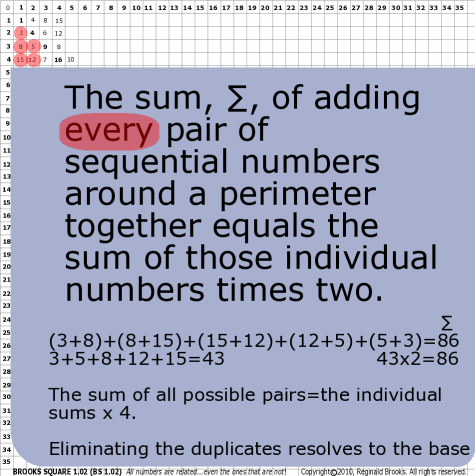~click to enlarge image
61

BS Rule 61: Not strictly a rule of the matrix, just an obvious mathematical manipulation that is useful to remember, take any series of numbers, add them together, the sum, ∑, times 2 will equal the sums of all the pairs of those numbers in that group arranged along a perimeter. The sum, ∑, times 4 will equal the sums of all possible pair combinations of those numbers.

Note:

 numbers ∑ all x2 x4 ∑ perimeter pairs ∑ all perimeter pairs ∑ possible pairs ∑ all possible pairs 3, 8, 5, 15, 12 43 86 172 3+8=11 8+15=23 15+12=27 12+5=17 5+3=8 86 315=18 3+12=15 3+8=11 3+5=8 8+5=13 8+12=20 8+15=23 5+15=20 5+12=17 172 86 172 86 172

Triangles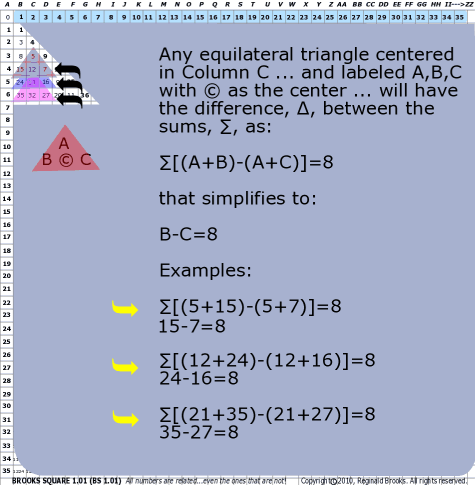~click to enlarge image 62 BS Rule 62: Any equilateral triangle formed in Column C, and labeled A (top), B, © and C, will have the difference, ∆, between the sums, ∑, A+B+© and A+C+© is 8. Simplified: B-C=8 Note: This follows from BS Rule 31, where the ∆ in number values from Column B to C=3, Column C to D=5, Column D to E=7,... and therefore, Column B to D=8 as the columns decrease in value by -1, -3, -5, ...left to right, respectively.

TOP ]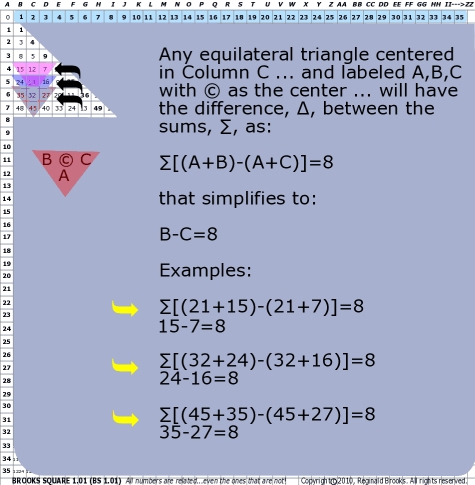~click to enlarge image 63 BS Rule 63: The same Rule 62 in reverse, A, ©, B and C (bottom) is true.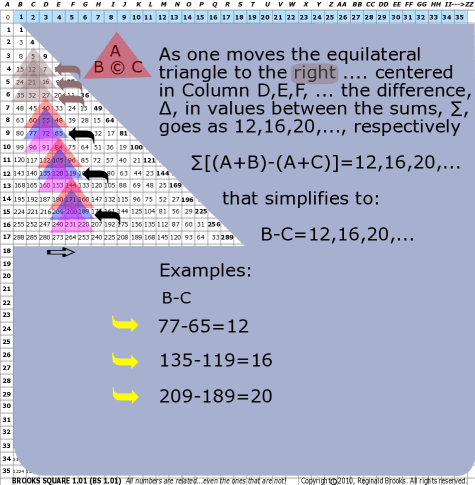~click to enlarge image 64 BS Rule 64: Following BS Rule 31 ... and similar to Rules 62 and 63 above ... as one moves the triangle to the right, Columns D, E, F,... the difference, ∆, in values goes as: 12, 16, 20, ... Note: C to E=12, D to F=16, E to G=20, ... and so on.

TOP ]

Square and Rectangles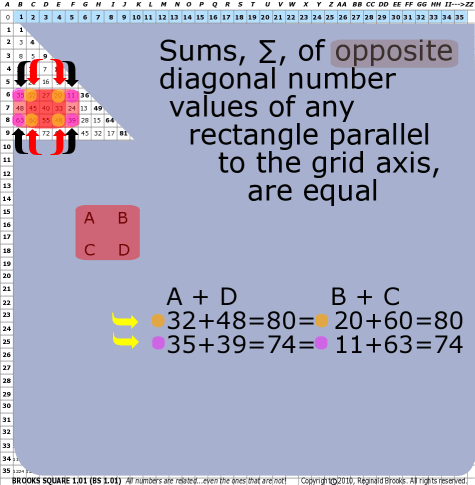~click to enlarge image
65

BS Rule 65: Additions of opposite diagonal number values of any rectangle parallel to the grid axis, are equal.

Note:

 A + D B + C 32+48=80 20+60=80 35+39=74 11+63=74

TOP ]

Diamond-Squares

 TAOST: Rules 1-50 | Rules 51-80 | Rules 81-99 | Rules 100-107 | Rules 108-153 | TCAOP: Rule 154 | Rules 155-157 | Rules 158-159 | Rule 160 | Interconnectedness: Rules 161-175 | Appendix A: Rules 176-181 | Appendix B: Rules 182-200 |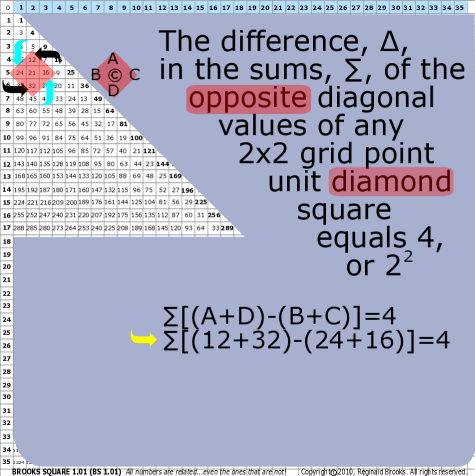~click to enlarge image
66

BS Rule 66: The difference, ∆, in the sums, ∑, of the opposite diagonal values of any 2x2 grid point unit diamond-squares = 4, or 22

Note:

 ∑(A + D) - ∑(B + C) ∆ 12+32 24+16 4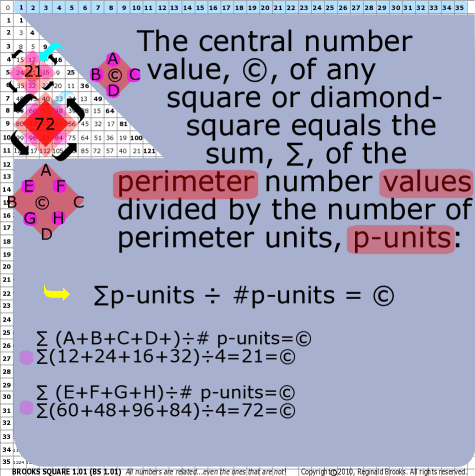~click to enlarge image
67

BS Rule 67: The central number value, ©, of any square or diamond-square equals the sum, ∑, of the perimeter number values divided by the number (quantity, #) of perimeter units, p-units.

Note: ∑p-units ÷ #p-units = ©

 ∑p-units ÷ #p-units © A+B+C+D 4 21 12+24+16+32 4 21 E+F+G+H 4 72 60+48+96+84 4 72

TOP ]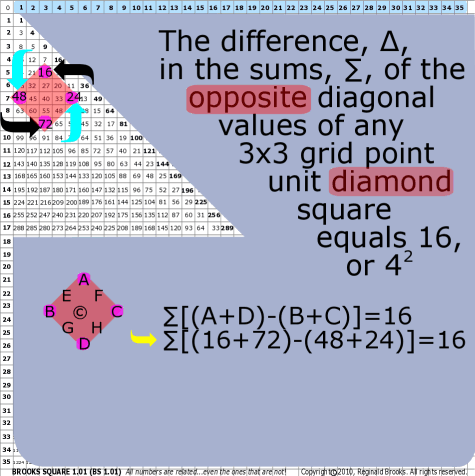~click to enlarge image
68

BS Rule 68: The difference, ∆, in the sums, ∑, of the opposite diagonal number values of any 3x3 grid point unit diamond-squares = 16, or 42.

Note:

 ∑(A + D) - ∑(B + C) ∆ 16+72 48+24 16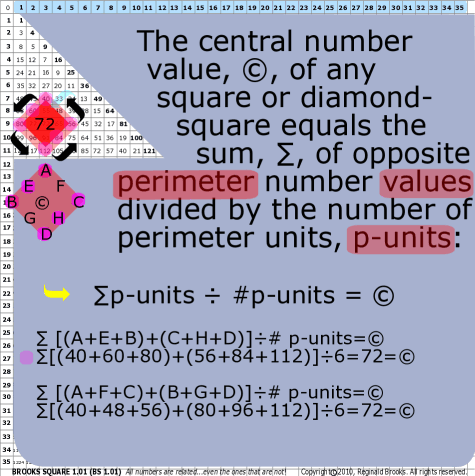~click to enlarge image
69

BS Rule 69: The central number value, ©, of any square or diamond-square equals the sum, ∑, of opposite side perimeter number values divided by the number (quantity, #) of perimeter units, p-units.

Note: ∑p-units ÷ #p-units = ©

 ∑p-units ÷ #p-units © (A+E+B) +(C+H+D) 6 72 (40+60+80) +(56+84+112) 6 72 (A+F+C) +(B+G+D) 6 72 (40+48+56) +(80+96+112) 6 72

TOP ]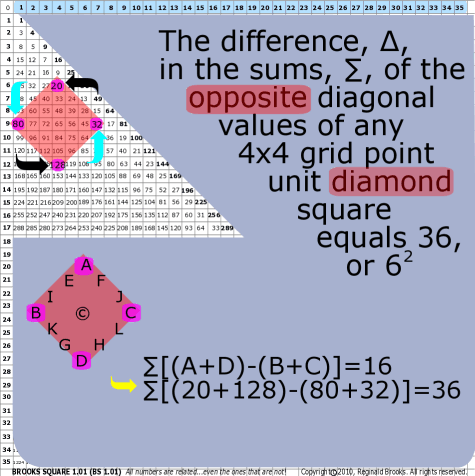~click to enlarge image
70

BS Rule 70: The difference, ∆, in the sums, ∑, of the opposite diagonal number values of any 4x4 grid point unit diamond-squares = 36, or 62.

Note:

 ∑(A + D) - ∑(B + C) ∆ 20+128 80+32 36

TOP ]

 TAOST: Rules 1-50 | Rules 51-80 | Rules 81-99 | Rules 100-107 | Rules 108-153 | TCAOP: Rule 154 | Rules 155-157 | Rules 158-159 | Rule 160 | Interconnectedness: Rules 161-175 | Appendix A: Rules 176-181 | Appendix B: Rules 182-200 |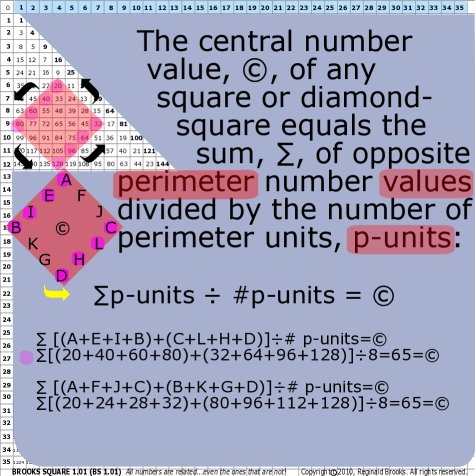~click to enlarge image
71

BS Rule 71: The central number value, ©, of any square or diamond-square equals the sum, ∑, of opposite side perimeter number values divided by the number (quantity, #) of perimeter units, p-units.

Note: ∑p-units ÷ #p-units = ©

 ∑p-units ÷ #p-units © (A+E+I+B) +(C+L+H+D) 8 65 (20+40+60+80) +(32+64+96+128) 8 65 (A+F+J+C) +(B+K+G+D) 8 65 (20+24+28+32) +(80+96+112+128) 8 65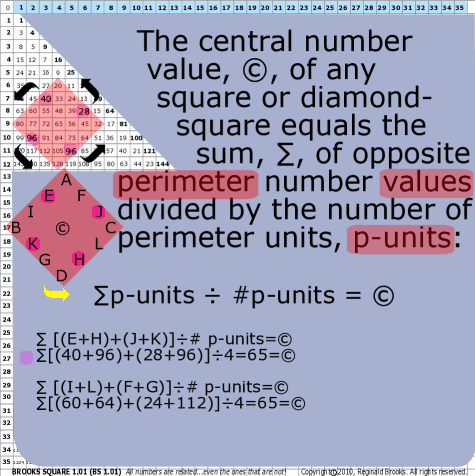~click to enlarge image
72

BS Rule 72: The central number value, ©, of any square or diamond-square equals the sum, ∑, of symmetrically opposite individual perimeter number values divided by the number (quantity, #) of perimeter units, p-units.

Note: ∑p-units ÷ #p-units = ©

 ∑p-units ÷ #p-units © (E+H)+(J+K) 4 65 (40+96)+(28+96) 4 65 (I+L)+(F+G) 4 65 (60+64)+(24+112) 4 65

TOP ]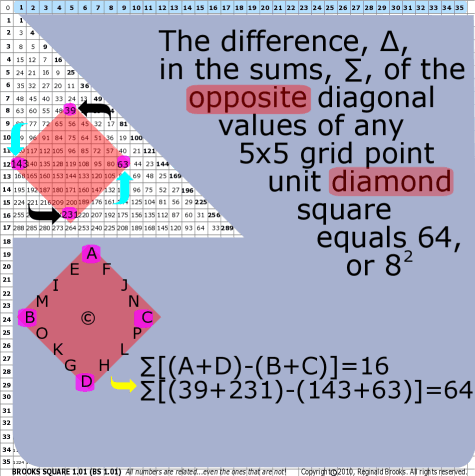~click to enlarge image
73

BS Rule 73: The difference, ∆, in the sums, ∑, of the opposite diagonal number values of any 5x5 grid point unit diamond-squares = 64, or 82, and so on.

Note:

 ∑(A + D) - ∑(B + C) ∆ 39+231 143+63 64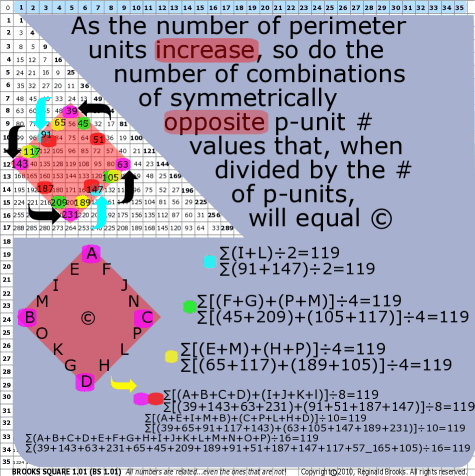~click to enlarge image
74

BS Rule 74: As in Rules 69, 71, and 72, as the number of perimeter units increase, so do the number of combinations of symmetrically opposite p-unit number values that, when divided by the # of p-units, will = ©.

Note:

 ∑p-units ÷ #p-units © I+L 2 119 F+G+P+M 4 119 (E+M)+(H+P) 4 119 (A+B+C+D) +(I+J+K+L) 8 119 (A+E+I+M+B) +(C+P+L+H+D) 10 119 A+B+C+D+E+F+G+H+I+J+K+L+M+N+O+P 16 119

TOP ]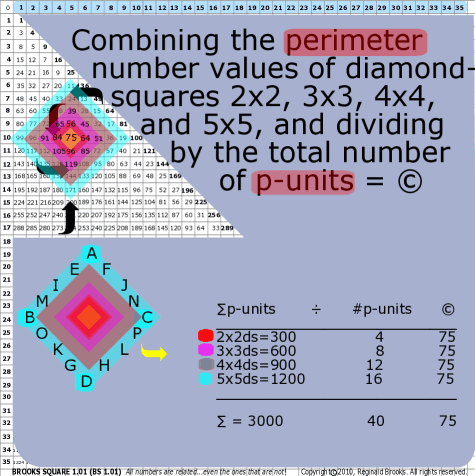~click to enlarge image
75

BS Rule 75: Combining the perimeter number values of diamond-squares 2x2, 3x3, 4x4,and 5x5, and dividing by the total number,#, of p-units = ©.

Note: ∑p-units ÷ #p-units = ©

 ∑p-units ÷ #p-units © 2x2ds=300 4 75 3x3ds=600 8 75 4x4ds=900 12 75 5x5ds=1200 16 75 ____________ ____ ∑ = 3000 40 75

TOP ]

 TAOST: Rules 1-50 | Rules 51-80 | Rules 81-99 | Rules 100-107 | Rules 108-153 | TCAOP: Rule 154 | Rules 155-157 | Rules 158-159 | Rule 160 | Interconnectedness: Rules 161-175 | Appendix A: Rules 176-181 | Appendix B: Rules 182-200 |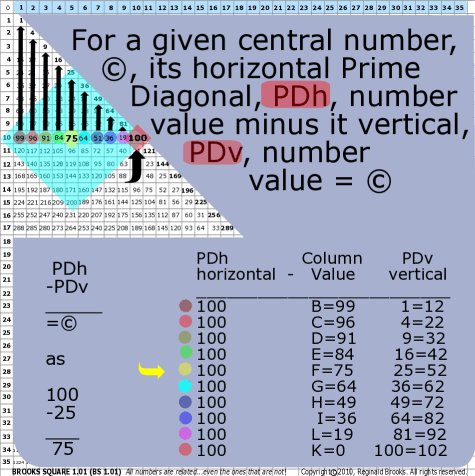~click to enlarge image
76

BS Rule 76: For a given central number, ©, its horizontal Prime Diagonal, PDh, number value minus it vertical, PDv, number value = ©.

Note: PDh-PDv=© as 100-25=75  Restated: PDh-Column Value=PDv

 PDh horizontal - Column Value PDv vertical 100 B=99 1=12 100 C=96 4=22 100 D=91 9=32 100 E=84 16=42 100 F=75 25=52 100 G=64 36=62 100 H=49 49=72 100 I=36 64=82 100 L=19 81=92 100 K=0 100=102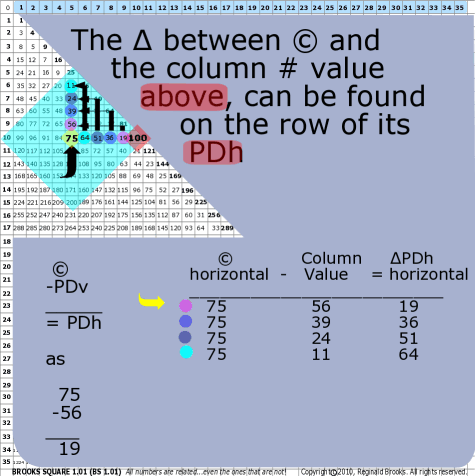~click to enlarge image
77

BS Rule 77: For a given central number, ©, the difference,∆, between it and the 1st vertical number value above it is found on the 1st Diagonal intersecting that row.

For a given central number, ©, the difference,∆, between it and the 2nd vertical number value above it is found on the 2nd Diagonal intersecting that rowr.

For a given central number, ©, the difference,∆, between it and the 3rd vertical number value above it is found on the 3rd Diagonal intersecting that row, and so on.

Note: Restated: The ∆ between © and the column # value above, can be found on the row of its PDh.

 © horizontal - Column Value ∆ PDh horizontal 75 56 19 75 39 36 75 24 51 75 11 64

TOP ]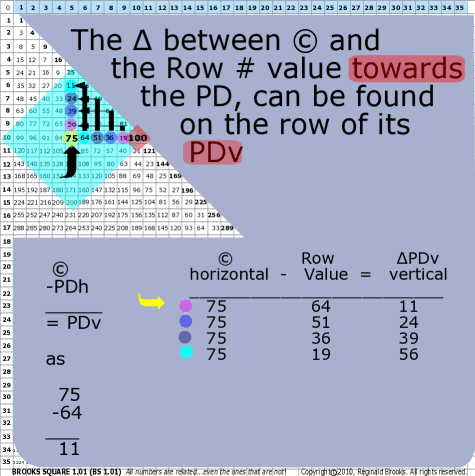~click to enlarge image
78

BS Rule 78: Similarly, for a given central number, ©, the difference, ∆, between it and the 1st horizontal row number towards the PD is found vertically in the column above © on the 1st Diagonal intersecting that column.

For a given central number, ©, the difference, ∆, between it and the 2nd horizontal row number towards the PD is found vertically in the column above © on the 2nd Diagonal intersecting that column.

For a given central number, ©, the difference, ∆, between it and the 3rd horizontal row number towards the PD is found vertically in the column above © on the 3rd Diagonal intersecting that column, and so on.

Note: Restated: The ∆ between © and the row # towards the PD, can be found on the column of its PDv.

 © horizontal - Row Value ∆ PDv vertical 75 64 11 75 51 24 75 36 39 75 19 56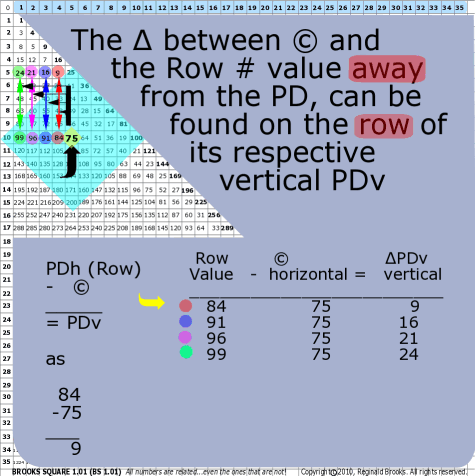~click to enlarge image
79

BS Rule 79: Similarly, for a given central number, ©, the difference, ∆, between it and the 1st horizontal row number away from the PD is found vertically in the column above that 1st horizontal row # on the 1st Diagonal intersecting that column.

For a given central number, ©, the difference, ∆, between it and the 2nd horizontal row number away from the PD is found vertically in the column above that 2nd horizontal row # on the 2nd Diagonal intersecting that column.

For a given central number, ©, the difference, ∆, between it and the 3rd horizontal row number away from the PD is found vertically in the column above that 3rd horizontal row # on the 3rd Diagonal intersecting that column, and so on.

Note: Restated: The ∆ between © and the row # away from the PD, can be found on the row of its respective vertical PDv.

 Row  value - © horizontal ∆ PDv vertical 84 75 9 91 75 16 96 75 21 99 75 24

TOP ]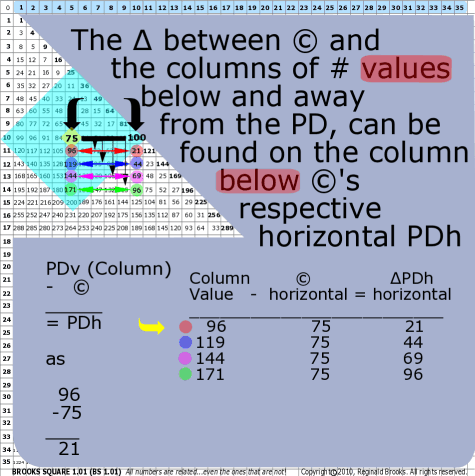~click to enlarge image
80

BS Rule 80: Similarly, for a given central number, ©, the difference, ∆, between it and the 1st vertical column number below, away from the PD is found horizontally in the row where the the 1st Diagonal intersects.

For a given central number, ©, the difference, ∆, between it and the 2nd vertical column number below, away from the PD is found horizontally in the row where the the 2nd Diagonal intersects.

For a given central number, ©, the difference, ∆, between it and the 3rd vertical column number below, away from the PD is found horizontally in the row where the the 3rd Diagonal intersects.

Note: Restated: The ∆ between © and the column of #s below, away from the PD, can be found on the column below ©’s respective horizontal PDh.

 Column value - © horizontal ∆ PDh vertical 96 75 21 119 75 44 144 75 69 171 75 96

TOP ]

 TAOST: Rules 1-50 | Rules 51-80 | Rules 81-99 | Rules 100-107 | Rules 108-153 | TCAOP: Rule 154 | Rules 155-157 | Rules 158-159 | Rule 160 | Interconnectedness: Rules 161-175 | Appendix A: Rules 176-181 | Appendix B: Rules 182-200 |

NEXT: On to I. TAOST>IC. Geometrics-relationships- Brooks (Base) Square

Back to I. TAOST>IA. Geometrics-lines - Brooks (Base) Square

Page 2a- PIN: Pattern in Number...from primes to DNA.

Page 2b- PIN: Butterfly Primes...let the beauty seep in..

Page 2c- PIN: Butterfly Prime Directive...metamorphosis.

Page 2d- PIN: Butterfly Prime Determinant Number Array (DNA) ~conspicuous abstinence~.

Page 3- GoDNA: the Geometry of DNA (axial view) revealed.

Page 4- SCoDNA: the Structure and Chemistry of DNA (axial view).

Page 5a- Dark-Dark-Light: Dark Matter = Dark Energy

Page 5b- The History of the Universe in Scalar Graphics

Page 5c- The History of the Universe_update: The Big Void

Page 6a- Geometry- Layout

Page 6b- Geometry- Space Or Time Area (SOTA)

Page 6c- Geometry- Space-Time Interactional Dimensions(STID)

Page 6d- Distillation of SI units into ST dimensions

Page 6e- Distillation of SI quantities into ST dimensions

Page 7- The LUFE Matrix Supplement: Examples and Proofs: Introduction-Layout & Rules

Page 7c- The LUFE Matrix Supplement: References

Page 8a- The LUFE Matrix: Infinite Dimensions

Page 9- The LUFE Matrix:E=mc2

Page 10- Quantum Gravity ...by the book

Page 11- Conservation of SpaceTime

Page 12- LUFE: The Layman's Unified Field Expose`

Page 13- GoMAS: The Geometry of Music, Art and Structure ...linking science, art and esthetics. Part I

Page 14- GoMAS: The Geometry of Music, Art and Structure ...linking science, art and esthetics. Part II

Page 15- Brooks (Base) Square (BS): The Architecture of Space-Time (TAOST) and The Conspicuous Absence of Primes (TCAOP) - a brief introduction to the series

Page 16- Brooks (Base) Square interactive (BBSi) matrix: Part I "BASICS"- a step by step, multi-media interactive

Page 17- The Architecture Of SpaceTime (TAOST) as defined by the Brooks (Base) Square matrix and the Inverse Square Law (ISL).